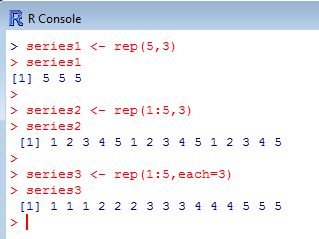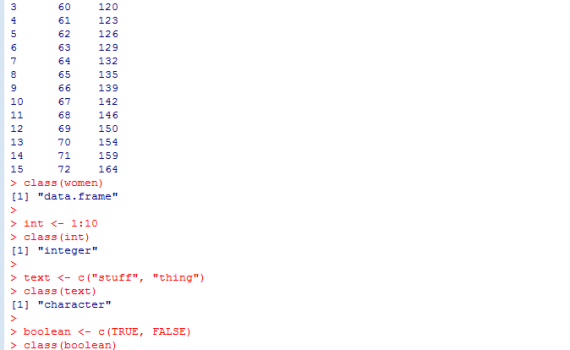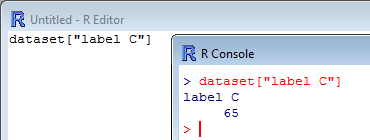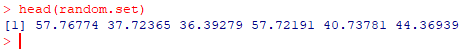R -6- Useful functions

This section provides you few a few useful or frequently used functions that may make your work quicker or at least easier. Making series, handling vectors and objects…Here you will find useful functions to create series of numbers to populate your tables and objects. These functions are also quite useful when you want to make a simulation or try functions on a random data set or on a test sample. Note that some functions return integers (numbers […]

1. Creating seriesHere are a few functions which are useful to handle and manage objects. ls() lists all the vectors and variables currently stored in memory. [code language=”r”] ls() [/code] Note that the function objects() does exactly the same.   rm() erases from the memory the vector or variable which is named […]

2. Managing objectshead(test) returns by default the first sixth entries in the vector “test”; head(test, 3) returns only the first three entries in the vector “test”. Check the example below: [code language=”r”] test <- c(4,7,9,6,89,45,3,5,78,23,45,0,2,12) test head(test) head(test,  3) [/code]   tail(test) returns the last sixth entries in the vector “test”; tail(test, […]

3. Diverse functionsThe function rnorm() generates automatically a series of random values which are normally distributed. This comes quite handy when you want to test functions, statistical analyses or scripts on a random dataset, and you need to be sure that the content is normally distributed beforehand. rnorm() needs the following information: […]